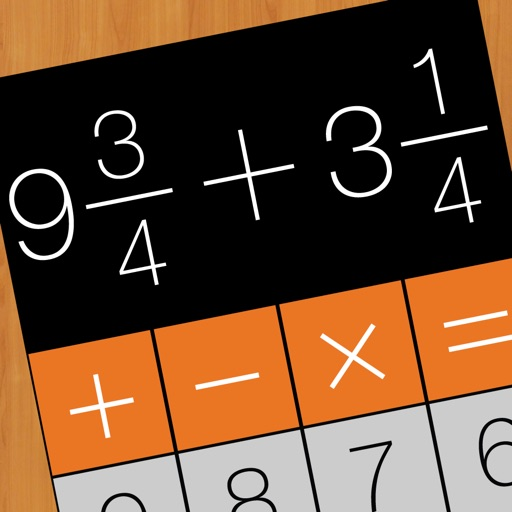## iFraction is a calculator for fraction math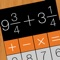# iFraction Calculator Plus

by CONG DUC TRAN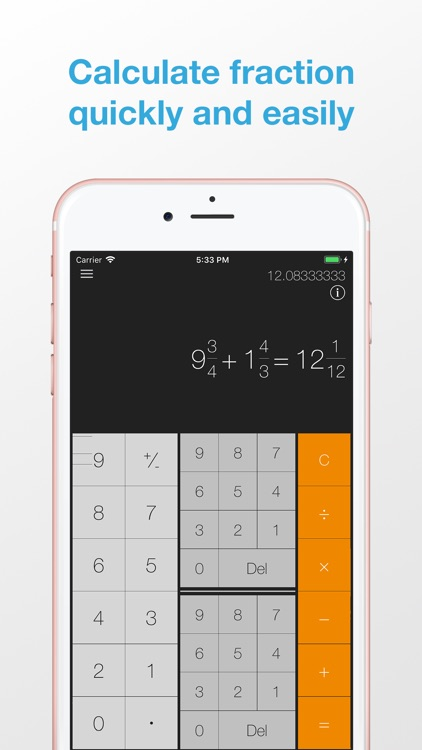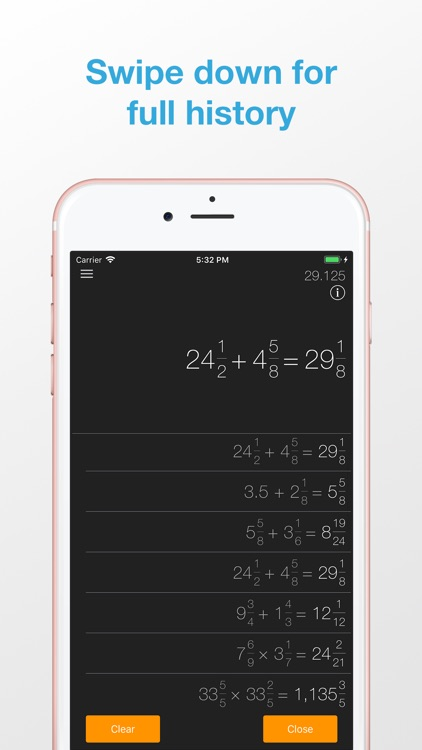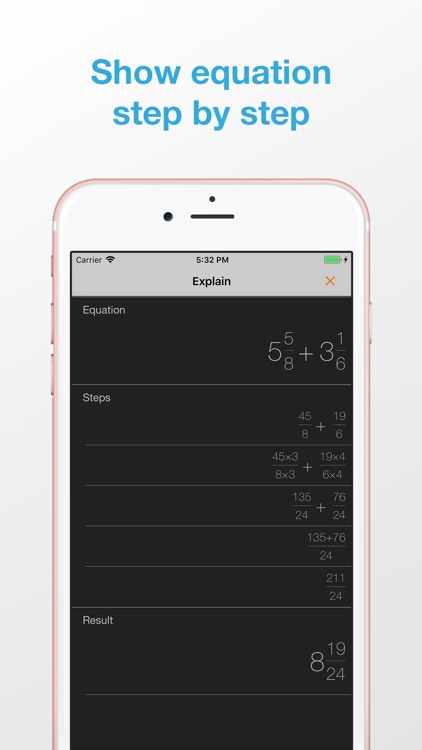iFraction is a calculator for fraction math. This app aims to help you to deal with everyday fraction problems.### App Details

Version
2.0
Rating
(15)
Size
12Mb
Genre
Productivity Utilities
Last updated
September 24, 2018
Release date
April 2, 2017

### App Screenshots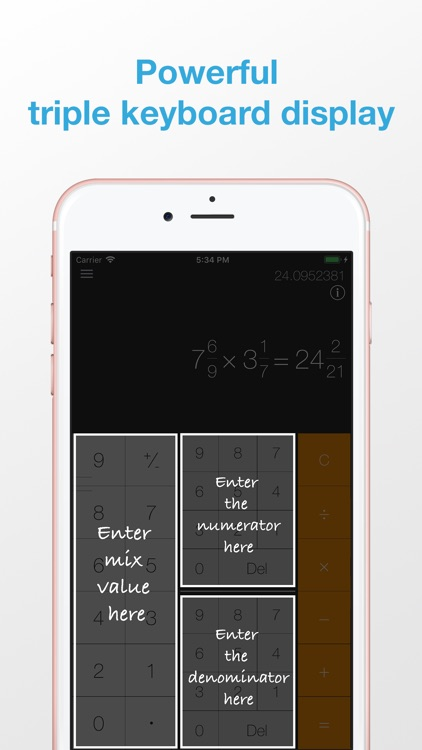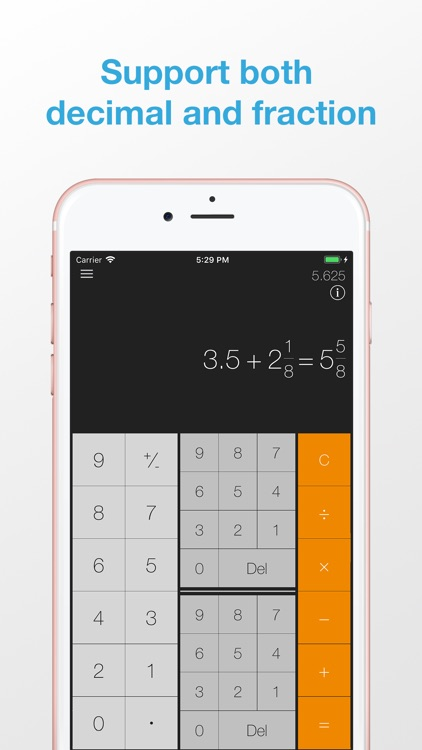### App Store Description

iFraction is a calculator for fraction math. This app aims to help you to deal with everyday fraction problems.

Key feature:
- Compute fraction math (addition, subtraction, multiplication, division)
- Calculate both decimals and fractions in one calculator
- Convert decimal number to fraction and vice versa